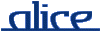# alice library manual.# The Fn structure

## ________ Synopsis ____________________________________________________

```    signature FN
structure Fn : FN
```

The structure Fn defines basic generic combinators for functions that are useful for a wide range of programming tasks.

See also: General

## ________ Import ______________________________________________________

Imported implicitly.

## ________ Interface ___________________________________________________

```    signature FN =
sig
val id :      'a -> 'a
val const :   'a -> 'b -> 'a
val apply :   ('a -> 'b) * 'a -> 'b
val o :       ('b -> 'c) * ('a -> 'b) -> ('a -> 'c)
val curry :   ('a * 'b -> 'c) -> ('a -> 'b -> 'c)
val uncurry : ('a -> 'b -> 'c) -> ('a * 'b -> 'c)
val flip :    ('a * 'b -> 'c) -> ('b * 'a -> 'c)
val repeat :  int -> ('a -> 'a) -> ('a -> 'a)
val forever : ('a -> 'a) -> 'a -> 'b
val iter :    int -> (unit -> unit) -> unit
end

________ Description _________________________________________________

Items not described here are as in the Standard ML Basis'
General structure.

val id : 'a -> 'a

id is the identity function. Thus, id a
is equivalent to a.

val const : 'a -> 'b -> 'a

const a is a constant function that always returns
a. Thus, const a b is equivalent to
a, except for side-effects.

val apply : ('a -> 'b) * 'a -> 'b

apply(f,a) applies f to
a. Thus, it is equivalent to f a.

val o : ('b -> 'c) * ('a -> 'b) -> ('a -> 'c)

f o g is the function composition of
f
and g. Thus, (f o g) a is
equivalent to f(g a).

val curry : ('a * 'b -> 'c) -> ('a -> 'b -> 'c)

curry f transforms the binary function
f into curried form. Thus, curry f a b
is equivalent to f(a,b).

val uncurry : ('a -> 'b -> 'c) -> ('a * 'b -> 'c)

uncurry f transforms a curried function
f into a binary function. Thus, uncurry f
(a,b) is equivalent to f a b.

val flip : ('a * 'b -> 'c) -> ('b * 'a -> 'c)

flip f switches the argument order of the binary
function
f. Thus, flip f (a,b) is
equivalent to f(b,a).

val repeat : int -> ('a -> 'a) -> ('a -> 'a)

repeat n f is the n-fold composition
of f. Thus, repeat n f a is equivalent
to f(...(f(a))...),
where f occurs n times.

val forever : ('a -> 'a) -> 'a -> 'b

forever f a performs infinite repetition of the function
f. Thus, forever f can be thought of as
being equivalent to repeat ∞ f.

val iter : int -> (unit -> unit) -> unit

iter n f performs n times the
application f(). Thus, iter n f is
equivalent to (f();...;f()), with
f occurring n times.

last modified 2007/Mar/30 17:10

```